## Stack Abuse: Python List Sorting with sorted() and sort()

In this article, we’ll examine multiple ways to sort lists in Python.

Python ships with two built-in methods for sorting lists and other iterable objects. The method chosen for a particular use-case often depends on whether we want to sort a list in-place or return a new version of the sorted list.

Assuming we want to sort a list in place, we can use the `list.sort()` method as follows:

``>>> pets = ['Turtle', 'Cat', 'Fish', 'Dingo'] >>> pets.sort() >>> pets ['Cat', 'Dingo', 'Fish', 'Turtle'] ``

By default, the list is sorted in ascending order. Notice how the original pets list is changed after the sort method is called on it. If we don’t want this to happen, we can use the built-in `sorted()` function to return a new sorted list while leaving the original list unchanged:

``>>> pets = ['Turtle', 'Cat', 'Fish', 'Dingo'] >>> new_pets = sorted(pets) >>> new_pets ['Cat', 'Dingo', 'Fish', 'Turtle'] >>> pets ['Turtle', 'Cat', 'Fish', 'Dingo'] ``

The reverse argument can be used to sort lists in descending order:

``>>> pets = ['Turtle', 'Cat', 'Fish', 'Dingo'] >>> new_pets = sorted(pets, reverse=True) >>> new_pets ['Turtle', 'Fish', 'Dingo', 'Cat'] >>> pets.sort(reverse=True) >>> pets ['Turtle', 'Fish', 'Dingo', 'Cat'] ``

However, there are scenarios where we might want to sort a list based on custom criteria that we define. For example, we may want to sort our pets list by the length of each entry. In that case Python offers the key argument, which accepts a user-defined function to specify the sorting criteria:

``>>> pets = ['Turtle', 'Cat', 'Fish', 'Dingo'] >>> get_len(x): ...    return len(x) ... >>> new_pets = sorted(pets, key=get_len) >>> new_pets ['Cat', 'Fish', 'Dingo', 'Turtle'] >>> pets.sort(key=get_len) >>> pets ['Cat', 'Fish', 'Dingo', 'Turtle'] ``

Now let’s consider a slightly more complex example. Here we have a list of dictionaries that contain data about a group of people, and we want to sort the list based the peoples’ ages, in descending order. To do this we will use both the `key` and `reverse` keyword arguments, as well as a Python lambda function. That way we can create the sorting function on the fly, instead of defining it beforehand:

``>>> data = [ { 'name': 'Billy', 'age': 26, 'country': 'USA' }, { 'name': 'Timmy', 'age': 5, 'country': 'Australia' }, { 'name': 'Sally', 'age': 19, 'country': 'Costa Rica' }, { 'name': 'Tommy', 'age': 67, 'country': 'Serbia' } ] >>> new_data = sorted(data, key=lambda x: x['age'], reverse=True) >>> new_data [{'country': 'Serbia', 'age': 67, 'name': 'Tommy'}, {'country': 'USA', 'age': 26, 'name': 'Billy'}, {'country': 'Costa Rica', 'age': 19, 'name': 'Sally'}, {'country': 'Australia', 'age': 5, 'name': 'Timmy'}] >>> data.sort(key=lambda x: x['age'], reverse=True) >>> data [{'country': 'Serbia', 'age': 67, 'name': 'Tommy'}, {'country': 'USA', 'age': 26, 'name': 'Billy'}, {'country': 'Costa Rica', 'age': 19, 'name': 'Sally'}, {'country': 'Australia', 'age': 5, 'name': 'Timmy'}] ``

Notice how the dictionaries startd in a seamingly random order and then ended up with the oldest people first and youngest people last in the list.

Using the sorting functions and lambdas in this way allows us to easily sort complex data structures, all in one line of code. And the order of the sort can be set to descending order by setting `reverse=True`.

This article was written by Jacob Stopak, a software consultant and developer with a passion for helping others improve their lives through code. Jacob is the creator of Code Card – a convenient tool for developers to look up, copy, and paste common code snippets.

Planet Python

## Stack Abuse: Python’s Bokeh Library for Interactive Data Visualization

### Introduction

In this tutorial, we’re going to learn how to use Bokeh library in Python. Most of you would have heard of matplotlib, numpy, seaborn, etc. as they are very popular python libraries for graphics and visualizations. What distinguishes Bokeh from these libraries is that it allows dynamic visualization, which is supported by modern browsers (because it renders graphics using JS and HTML), and hence can be used for web applications with a very high level of interactivity.

Bokeh is available in R and Scala language as well; however, its Python counterpart is more commonly used than others.

### Installation

The easiest way to install Boken using Python is through pip package manager. If you have pip installed in your system, run the following command to download and install Bokeh:

``\$   pip install bokeh ``

Note: If you choose this method of installation, you need to have numpy installed in your system already

Another method to install Bokeh is through Anaconda distribution. Simply go to your terminal or command prompt and run this command:

``\$   conda install bokeh ``

After completing this step, run the following command to ensure that your installation was successful:

``\$   bokeh --version ``

If the above command runs successfully i.e. the version gets printed, then you can go ahead and use bokeh library in your programs.

### Coding Exercises

In this part, we will be doing some hands-on examples by calling Bokeh library’s functions to create interactive visualizations. Let’s start by trying to make a square.

Note: Comments in the codes throughout this article are very important; they will not only explain the code but also convey other meaningful information. Furthermore, there might be ‘alternative’ or additional functionality that would be commented out, but you can try running it by uncommenting those lines.

#### Plotting Basic Shapes

Here we specify the `x` and `y` coordinates for points, which will be followed in sequence when the line is being drawn. The `figure` function instantiates a `figure` object, which stores the configurations of the graph you wish to plot. Here we can specify both the X range and Y range of the graph, which we set from 0 to 4, which covers the range of our data. The `line` method then draws a line between our coordinates, which is in the shape of a square.

``from bokeh.io import output_file, output_notebook   from bokeh.plotting import figure, show  x = [1, 3, 3, 1, 1]   y = [1, 1, 3, 3, 1]  # Display the output in a separate HTML file  output_file('Square.html', title='Square in Bokeh')   #output_notebook() # Uncomment this line to use iPython notebook  square = figure(title='Square Shape',                plot_height=300, plot_width=300,              x_range=(0, 4), y_range=(0, 4))  # Draw a line using our data square.line(x, y)   #square.circle(x, y) # Uncomment this line to add a circle mark on each coordinate  # Show plot show(square)   ``

You may have noticed in the code that there is an alternative to the `output_file` function, which would instead show the result in a Jupyter notebook by using the `output_notebook` function. If you’d prefer to use a notebook then replace the `output_file` function with `output_notebook` in the code throughout this article.

When you run the above script, you should see the following square opening in a new tab of your default browser.

Output: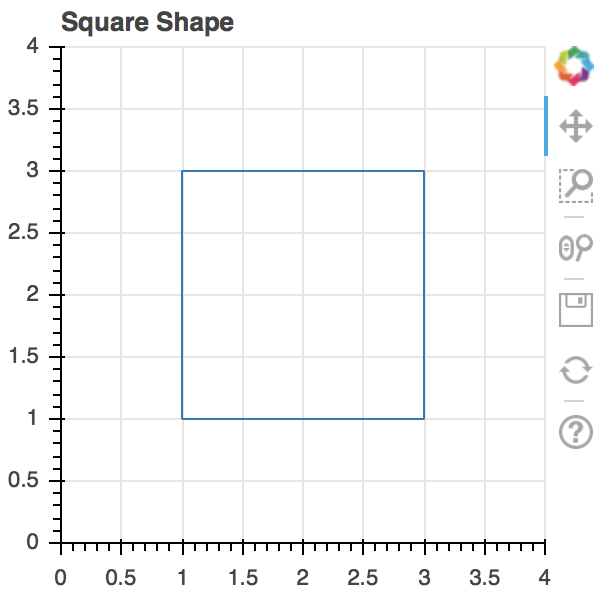In the image above, you can see the tools on the right side (pan, box zoom, wheel zoom, save, reset, help – from top to bottom); these tools enable you to interact with the graph.

Another important thing which will come in handy is that after every call to the “show” function if you create a new “figure” object, a subsequent call to the “show” function with the new figure passed as an argument would generate an error. To resolve that error, run the following code:

``from bokeh.plotting import reset_output  reset_output()   ``

The `reset_output` method resets the figure ID that the `show` function currently holds so that a new one can be assigned to it.

What we’ve done so far is rather basic, let’s now try to make multiple lines/map equations in a single graph. The most basic example for that would be to try and draw lines for the equations `y = x`, `y = x^2`, and `y = x^3`. So let’s see how we can make a graph to display them all at once using Bokeh:

``from bokeh.plotting import figure, output_file, show  # Declare data for our three lines x = [1, 2, 3, 4, 5, 6]   x_square = [i**2 for i in x]   x_cube = [i**3 for i in x]  # Declare HTML file as output for when show is called output_file("Eqs.html")  lines = figure(title='Line Comparisons', x_range=(0, 8), y_range=(0,100),      x_axis_label='X-Axis', y_axis_label='Y-Axis')   lines.line(x, x, legend="y = x", line_width=3) # Line for the equation y=x   lines.square(x, x, legend="y = x", size=10) # Add square boxes on each point on the line  lines.line(x, x_square, legend="y = x^2", line_width=3) #Line for the equation y=x^2   lines.circle(x, x_square, legend="y = x^2", size=10) # Add circles to points since it partially overlaps with y=x  lines.line(x, x_cube, legend="y = x^3", line_width=3) # Line for the equation y=x^3   lines.square(x, x_cube, legend="y = x^2", size=10) # Add square boxes on each point of the line  # Display the graph show(lines)   ``

Output: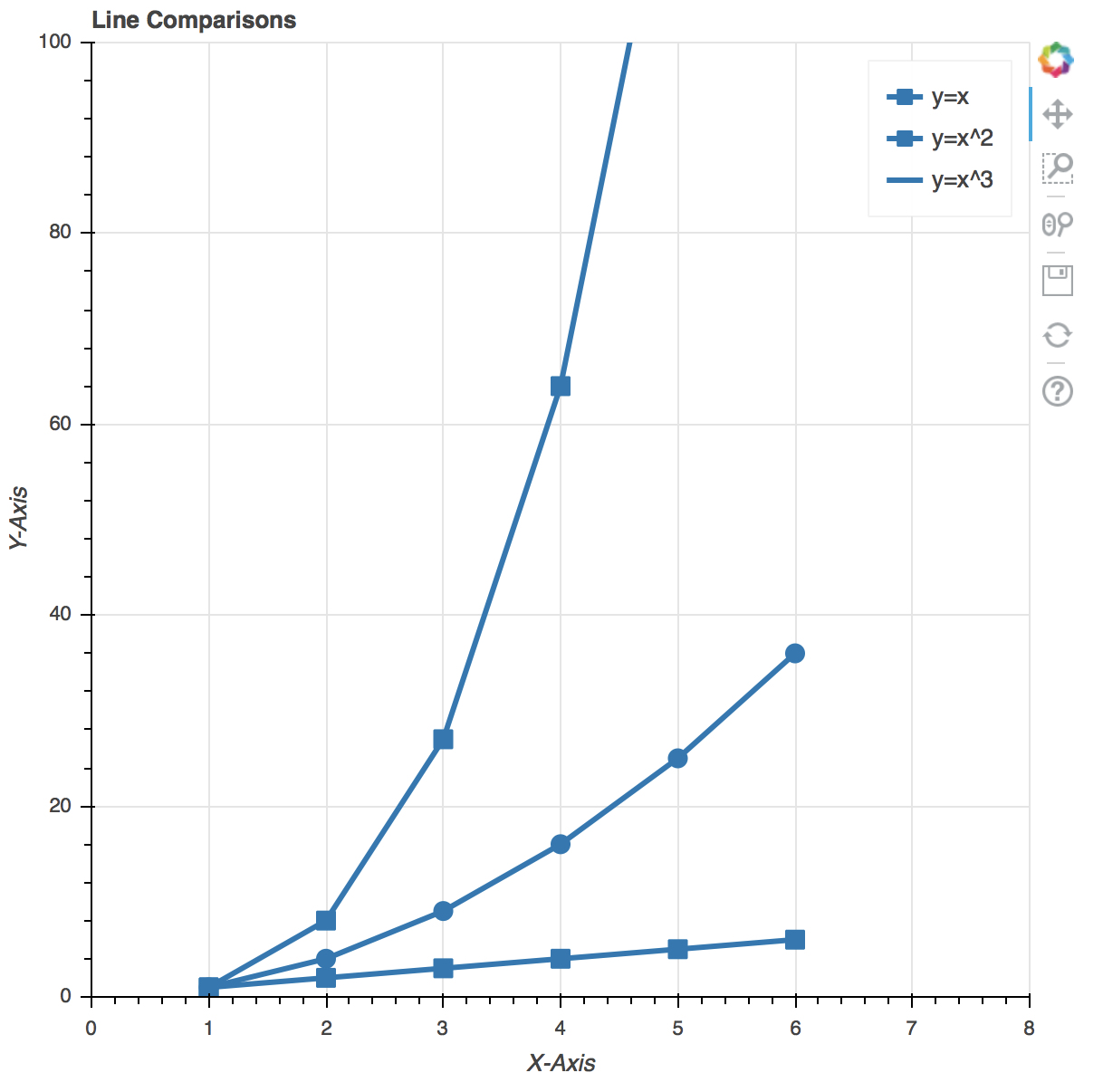Before we continue to plot a few more graphics, let’s first learn a few cool tricks to make your graphics more interactive, as well as aesthetic. For that we’ll first of all learn about the different tools that the Bokeh Library uses apart from the ones that are displayed alongside (either on top or on the right side) the graph. The explanations will be provided in the comments of the code below:

``# Use the same plot data as above x = [1, 2, 3, 4, 5, 6]   x_square = [i**2 for i in x]   x_cube = [i**3 for i in x]  #now let's make the necessary imports. Note that, in addition to the imports we made in the previous code, we'll be importing a few other things as well, which will be used to add more options in the 'toolset'.   # Same imports as before from bokeh.plotting import figure, output_file, show  # New imports to add more interactivity in our figures # Check out Bokeh's documentation for more tools (these are just two examples) from bokeh.models import HoverTool, BoxSelectTool  output_file("Eqs.html")  # Add the tools to this list tool_list = [HoverTool(), BoxSelectTool()]  # Use the tools keyword arg, otherwise the same lines = figure(title='Line Comparisons', x_range=(0, 8), y_range=(0, 100),      x_axis_label='X-Axis', y_axis_label='Y-Axis', tools=tool_list)  # The rest of the code below is the same as above lines.line(x, x, legend="y = x", line_width=3)   lines.square(x, x, legend="y = x", size=10)  lines.line(x, x_square, legend="y = x^2", line_width=3)   lines.circle(x, x_square, legend="y = x^2", size=10)  lines.line(x, x_cube, legend="y = x^3", line_width=3)   lines.square(x, x_cube, legend="y = x^2", size=10)  # Display the graph show(lines)   ``

Output: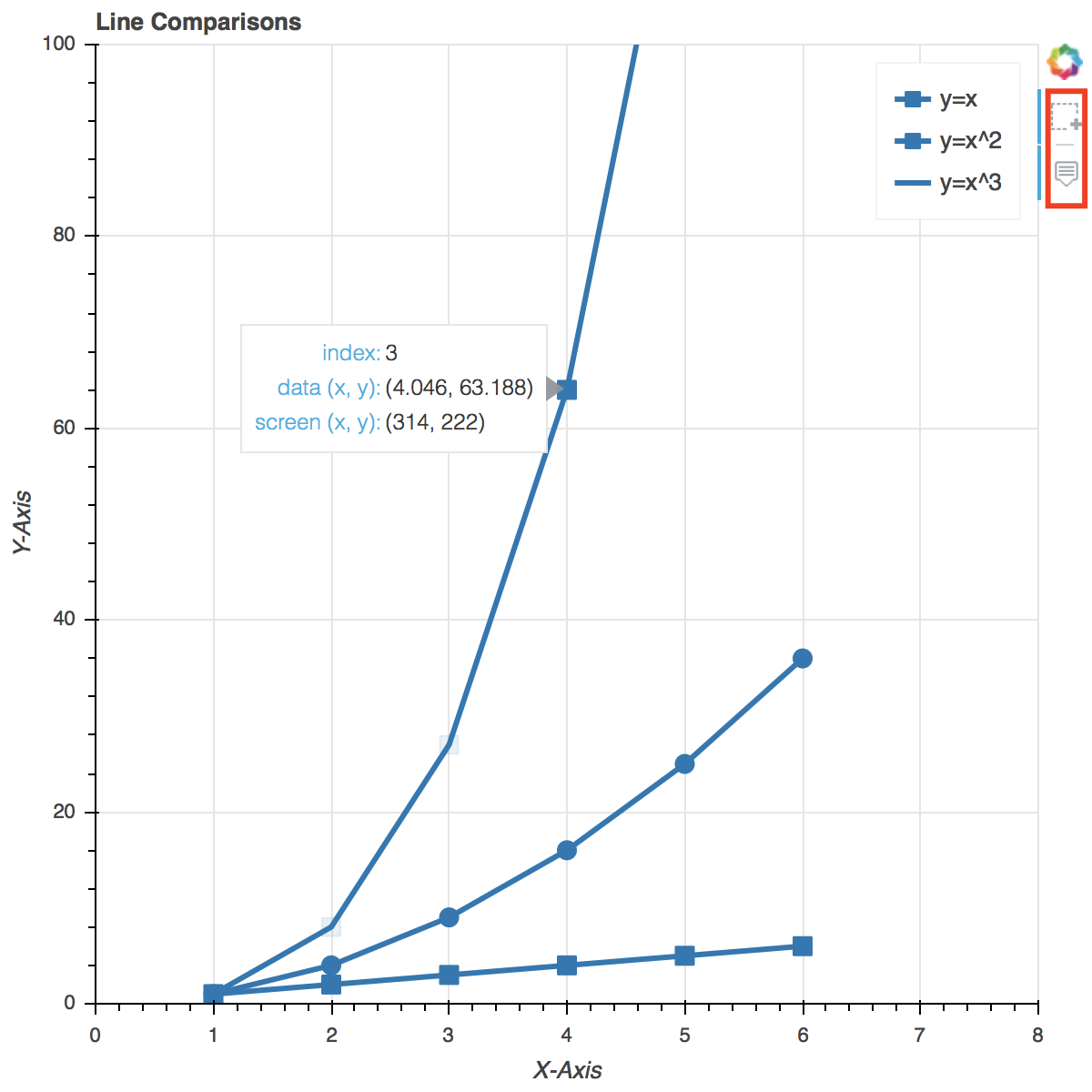In the above picture, you can see the two extra options added to the previously available tools. You can now also hover over any data point and its details will be shown, and you can also select a certain group of data points to highlight them.

#### Handling Categorical Data with Bokeh

Next thing that we’ll learn to do using Bokeh library is handling categorical data. For that, we’ll try and make a bar chart first. To make it interesting, let’s try and create a chart which represents the number of world cups won by Argentina, Brazil, Spain, and Portugal. Sounds interesting? Let’s code it.

``from bokeh.io import show, output_file   from bokeh. plotting import figure  output_file("cups.html")  # List of teams to be included in the chart. Add or # remove teams (and their World Cups won below) to # see how it affects the chart teams = ['Argentina', 'Brazil', 'Spain', 'Portugal']  # Activity: We experimented with the Hover Tool and the # Box Select tool in the previous example, try to # include those tools in this graph  # Number of world cups that the team has won wc_won = [5, 3, 4, 2]  # Setting toolbar_location=None and tools="" essentially # hides the toolbar from the graph barchart = figure(x_range=teams, plot_height=250, title="WC Counts",              toolbar_location=None, tools="")  barchart.vbar(x=teams, top=wc_won, width=0.5)  # Acitivity: Play with the width variable and see what # happens. In particular, try to set a value above 1 for # it   barchart.xgrid.grid_line_color = 'red'   barchart.y_range.start = 0  show(barchart)   ``

Output: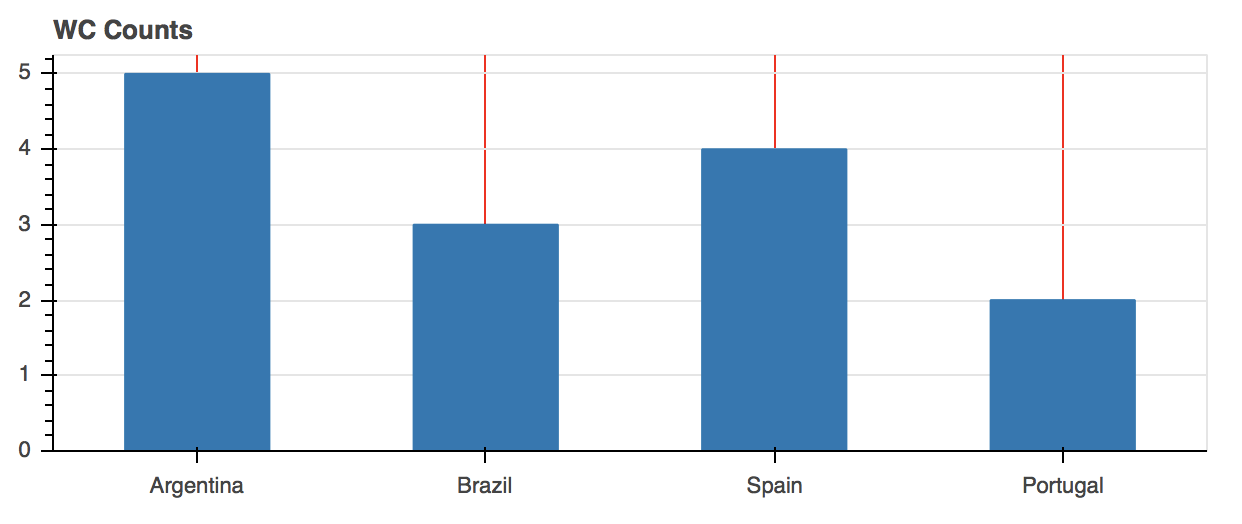Do you notice something in the graph above? It’s quite simple, and unimpressive, no? Let’s make some changes in the above code, and make it a bit more colorful and aesthetic. Bokeh has a lot of options to help us with that. Let’s see what we can do with it:

``# Mostly the same code as above, except with a few # additions to add more color to our currently dry graph  from bokeh.io import show, output_file   from bokeh.plotting import figure  # New imports below from bokeh.models import ColumnDataSource  # A was added 4 to the end of Spectral because we have 4 # teams. If you had more or less you would have used that # number instead from bokeh.palettes import Spectral4  from bokeh.transform import factor_cmap  output_file("cups.html")  teams = ['Argentina', 'Brazil', 'Spain', 'Portugal']   wc_won = [5, 3, 4, 2]  source = ColumnDataSource(data=dict(teams=teams, wc_won=wc_won, color=Spectral4))  barchart = figure(x_range=teams, y_range=(0,8), plot_height=250, title="World Cups Won",              toolbar_location=None, tools="")  barchart.vbar(x='teams', top='wc_won', width=0.5, color='color', legend='teams', source=source)   # Here we change the position of the legend in the graph # Normally it is displayed as a vertical list on the top # right. These settings change that to a horizontal list # instead, and display it at the top center of the graph barchart.legend.orientation = "horizontal"   barchart.legend.location = "top_center"  show(barchart)   ``

Output: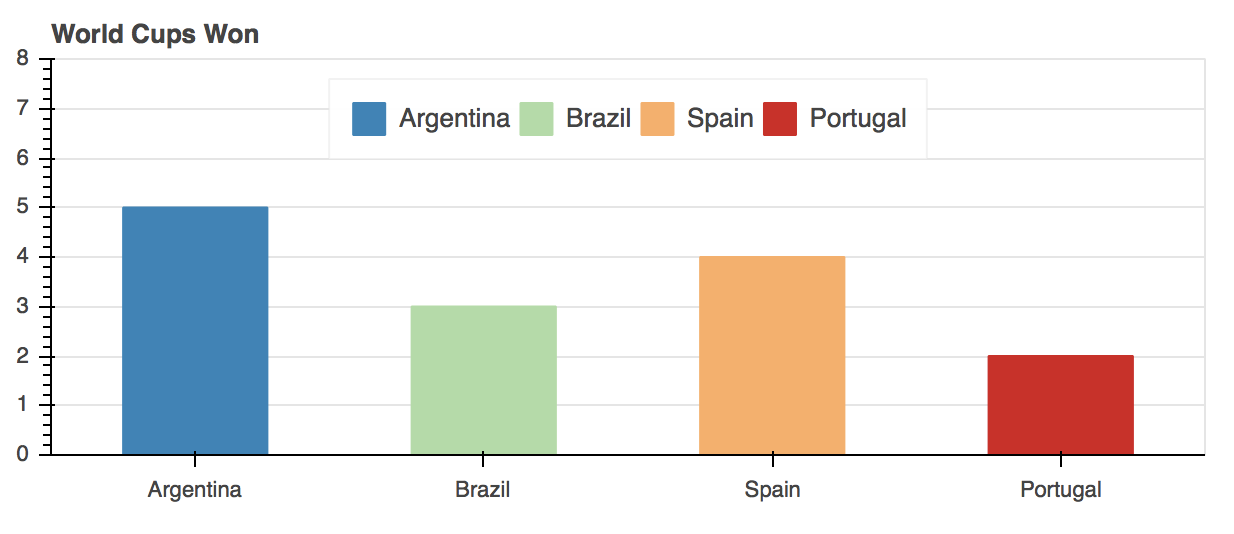Evidently, the new graph looks a lot better than before, with added interactivity.

Before concluding this article, I’d like to let you all know that this was just a glimpse of the functionality that Bokeh offers. There are tons of other cool things that you can do with it, and you should try them out by referring to Bokeh’s documentation and following the available examples.

### Conclusion

To sum it up, in this tutorial we learned about the Bokeh library’s Python variant. We saw how to download and install it using the `pip` or `anaconda` distribution. We used Bokeh library programs to make interactive and dynamic visualizations of different types and using different data types as well. We also learned, by seeing practical examples, the reason why Bokeh is needed even though there are other more popular visualization libraries like matplotlib and Seaborn available. In short, Bokeh is very resourceful and can pretty much do all kinds of interactive visualizations that you may want.

Planet Python

## Stack Abuse: The Python Help System

When writing and running your Python programs, you may get stuck and need to get help. You may need to know the meaning of certain modules, classes, functions, keywords, etc. The good news is that Python comes with an built-in help system. This means that you don’t have to seek help outside of Python itself.

In this article, you will learn how to use the built-in Python help system.

### Python help() function

This function helps us to get the documentation of a certain class, function, variable, module, etc. The function should be used on the Python console to get details of various Python objects.

#### Passing an Object to help() Function

The Python `help()` function has the following syntax:

``>>> help(object) ``

In the above syntax, the `object` parameter is the name of the object that you need to get help about.

For example, to know more about the Python’s `print` function, type the following command on the Python console:

``>>> help(print) ``

Output:

``Help on built-in function print in module builtins:  print(...)       print(value, ..., sep=' ', end='\n', file=sys.stdout, flush=False)      Prints the values to a stream, or to sys.stdout by default.     Optional keyword arguments:     file:  a file-like object (stream); defaults to the current sys.stdout.     sep:   string inserted between values, default a space.     end:   string appended after the last value, default a newline.     flush: whether to forcibly flush the stream. ``

To get help for the `dict` class, type the following on the Python console:

``>>> help(dict) ``

Output:

``Help on class dict in module builtins:  class dict(object)    |  dict() -> new empty dictionary  |  dict(mapping) -> new dictionary initialized from a mapping object's  |      (key, value) pairs  |  dict(iterable) -> new dictionary initialized as if via:  |      d = {}  |      for k, v in iterable:  |          d[k] = v  |  dict(**kwargs) -> new dictionary initialized with the name=value pairs  |      in the keyword argument list.  For example:  dict(one=1, two=2)  |    |  Methods defined here:  |    |  __contains__(self, key, /)  |      True if D has a key k, else False.  |    |  __delitem__(self, key, /)  |      Delete self[key].  |    |  __eq__(self, value, /)  |      Return self==value.  |    |  __ge__(self, value, /)  |      Return self>=value.  |    ... ``

You can also pass an actual list object to the `help()` function:

``>>> help(['a', 'b', 'c']) ``

Output:

``Help on list object:  class list(object)    |  list() -> new empty list  |  list(iterable) -> new list initialized from iterable's items  |    |  Methods defined here:  |    |  __add__(self, value, /)  |      Return self+value.  |    |  __contains__(self, key, /)  |      Return key in self.  |    |  __delitem__(self, key, /)  |      Delete self[key].  |    |  __eq__(self, value, /)  |      Return self==value.  |    |  __ge__(self, value, /)  |      Return self>=value.  |    |  __getattribute__(self, name, /)  |      Return getattr(self, name).  ... ``

We can see that when you pass an object to the `help()` function, it’s documentation or help page is printed. In the next section, you will learn about passing string arguments to the `help()` function.

#### Passing a String Argument to help()

If you pass a string as an argument, the string will be treated as the name of a function, module, keyword, method, class, or a documentation topic and the corresponding help page will be printed. To mark it as a string argument, enclose it within either single or double quotes.

For example:

``>>> help('print') ``

Output:

``Help on built-in function print in module builtins:  print(...)       print(value, ..., sep=' ', end='\n', file=sys.stdout, flush=False)      Prints the values to a stream, or to sys.stdout by default.     Optional keyword arguments:     file:  a file-like object (stream); defaults to the current sys.stdout.     sep:   string inserted between values, default a space.     end:   string appended after the last value, default a newline.     flush: whether to forcibly flush the stream. ``

Although we passed ‘print’ as a string argument, we still got the documentation for the Python’s `print` function. Here is another example:

``>>> help('def') ``

Output:

``Function definitions   ********************  A function definition defines a user-defined function object (see   section *The standard type hierarchy*):     funcdef        ::= [decorators] "def" funcname "(" [parameter_list] ")" ["->" expression] ":" suite    decorators     ::= decorator+    decorator      ::= "@" dotted_name ["(" [parameter_list [","]] ")"] NEWLINE    dotted_name    ::= identifier ("." identifier)*    parameter_list ::= (defparameter ",")*                       | "*" [parameter] ("," defparameter)* ["," "**" parameter]                       | "**" parameter                       | defparameter [","] )    parameter      ::= identifier [":" expression]    defparameter   ::= parameter ["=" expression]    funcname       ::= identifier  A function definition is an executable statement.  Its execution binds   the function name in the current local namespace to a function object   (a wrapper around the executable code for the function).  This  ... ``

Here we passed “def” as a string argument to the `help()` function and it returned the documentation for defining functions.

If no matching object, method, function, class or module is found, you will be notified. For example:

``>>> help('qwerty') ``

Output:

``No Python documentation found for 'qwerty'.   Use help() to get the interactive help utility.   Use help(str) for help on the str class.   ``

We are notified that no documentation was found for our string.

Sometimes, we may need to get help about a certain function that is defined in a certain Python library. This requires us to first import the library. A good example is when we need to get the documentation for the `log` function defined in Python’s `math` library. In this case, we first need to import the `math` library then we call the `help()` function as demonstrated below:

``>>> from math import log >>> help(log) ``

Output:

``Help on built-in function log in module math:  log(...)       log(x[, base])      Return the logarithm of x to the given base.     If the base not specified, returns the natural logarithm (base e) of x. ``

#### Using help() with no Argument

The `help()` function can be used without an argument. If you run the function without an argument, the interactive Python’s help utility will be started on the interpreter console. You just have to type the following command on the Python console:

``>>> help() ``

This will return the Python’s help utility on which you can type the name of the object you need to get help about. For example:

``help> print   ``

Output:

``Help on built-in function print in module builtins:  print(...)       print(value, ..., sep=' ', end='\n', file=sys.stdout, flush=False)      Prints the values to a stream, or to sys.stdout by default.     Optional keyword arguments:     file:  a file-like object (stream); defaults to the current sys.stdout.     sep:   string inserted between values, default a space.     end:   string appended after the last value, default a newline.     flush: whether to forcibly flush the stream. ``

Here is another example:

``help> return   ``

Output:

``The "return" statement   **********************     return_stmt ::= "return" [expression_list]  "return" may only occur syntactically nested in a function definition, not within a nested class definition.  If an expression list is present, it is evaluated, else "None" is   substituted.  "return" leaves the current function call with the expression list (or "None") as return value.  When "return" passes control out of a "try" statement with a "finally"   clause, that "finally" clause is executed before really leaving the   function.  In a generator function, the "return" statement indicates that the   generator is done and will cause "StopIteration" to be raised. The   returned value (if any) is used as an argument to construct   "StopIteration" and becomes the "StopIteration.value" attribute.  Related help topics: FUNCTIONS   ``

To leave the help utility and return to the Python console, just type “quit” and hit the enter key:

``help> quit   ``

Output:

``You are now leaving help and returning to the Python interpreter.   If you want to ask for help on a particular object directly from the   interpreter, you can type "help(object)".  Executing "help('string')"   has the same effect as typing a particular string at the help> prompt.   >>> ``

In the next section, we will be discussing how to define `help()` for our custom objects.

#### Defining Help Docs for Custom Functions and Classes

It is possible for us to define the output of `help()` function for our custom functions and classes by defining a docstring (document string). In Python, the first comment string added to the body of a method is treated as its docstring. The comment has to be surrounded with three double quotes. For example:

``def product(a, b):       """     This function multiplies two given integers, a and b     :param x: integer     :param y: integer     :returns: integer     """     return a * b ``

In the above example, we have defined a function named `product`. This function multiplies two integer values, `a` and `b` passed to it as arguments/parameters. See the comment enclosed within three double quotes:

``    """     This function multiplies two given integers, a and b     :param x: integer     :param y: integer     :returns: integer     """ ``

This will be treated as the docstring for the function `product`.

Now, create a new file and give it the name “myfile.py”. Add the following code to the file:

``def product(a, b):       """     This function multiplies two given integers, a and b     :param x: integer     :param y: integer     :returns: integer     """     return a * b  class Student:       """     Student class in Python. It will store student details     """     admission = 0     name = ''      def __init__(self, adm, n):         """         A constructor of the student object         :param adm: a positive integer,         :param n: a string         """         self.admission = adm         self.name = n ``

In the above example, a docstring has been defined for a function, class, and methods.

We now need to demonstrate how we can get the above docstring as the help documentation on our Python console.

First, we need to execute the script on the console in order to load both the function and class definition to the Python environment. We can use the Python’s `exec()` method for this. Run the following command on the Python console:

``>>> exec(open("myfile.py").read()) ``

Alternatively, if you have written the code within the Python IDLE, you simply have to run it.

We can now confirm whether the function and class modules have been detected by running the `globals()` command on the Python console:

``>>> globals() ``

In my case, I get the following output:

``{'__doc__': None, 'log': <built-in function log>, '__builtins__': <module 'builtins' (built-in)>, '__spec__': None, '__package__': None, '__name__': '__main__', '__loader__': <class '_frozen_importlib.BuiltinImporter'>, '__file__': 'C:/Users/admin/myfile.py', 'Student': <class '__main__.Student', 'product': <function product at 0x0000000003569B70>} ``

As shown in the above output, both `Student` and `product` are in the global scope dictionary. We can now use the `help()` function to get the help for the `Student` class and `product` function. Just run the following command on the Python console:

``>>> help('myfile') ``

Output:

``Help on module myfile:  NAME       myfile  CLASSES       builtins.object         Student      class Student(builtins.object)      |  Student class in Python. It will store student details      |        |  Methods defined here:      |        |  __init__(self, adm, n)      |      A constructor of the student object      |      :param adm: a positive integer,      |      :param n: a string      |        |  ----------------------------------------------------------------------      |  Data descriptors defined here:      |        |  __dict__      |      dictionary for instance variables (if defined)      |        |  __weakref__      |      list of weak references to the object (if defined)      |        |  ----------------------------------------------------------------------      |  Data and other attributes defined here:      |        |  admission = 0      |        |  name = ''  FUNCTIONS       product(a, b)         This function multiplies two given integers, a and b         :param x: integer         :param y: integer         :returns: integer  FILE       c:\users\admin\myfile.py ``

Let us check the help documentation for the `product` function:

``>>> help('myfile.product') ``

Output:

``Help on function product in myfile:  myfile.product = product(a, b)       This function multiplies two given integers, a and b     :param x: integer     :param y: integer     :returns: integer ``

Now, let us access the help documentation for the `Student` class:

``>>> help('myfile.Student') ``

Output:

``Help on class Student in myfile:  myfile.Student = class Student(builtins.object)    |  Student class in Python. It will store student details  |    |  Methods defined here:  |    |  __init__(self, adm, n)  |      A constructor of the student object  |      :param adm: a positive integer,  |      :param n: a string  |    |  ----------------------------------------------------------------------  |  Data descriptors defined here:  |    |  __dict__  |      dictionary for instance variables (if defined)  |    |  __weakref__  |      list of weak references to the object (if defined)  |    |  ----------------------------------------------------------------------  |  Data and other attributes defined here:  |    |  admission = 0  |    |  name = '' ``

In the output, we can see the documentation that we wrote for the `Student` class.

### Conclusion

Python comes with an built-in system from which we can get help in regards to modules, classes, functions, and keywords. This help utility can be accessed by the use of Python’s `help()` function in the REPL. When we call this function and pass an object to it, it returns the help page or the documentation for the object. When we run the function without an argument, the help utility is opened where we can get help about objects in an interactive way. Finally, to get help regarding our custom classes and functions, we can define docstrings.

Planet Python

## Stack Abuse: Python for NLP: Creating Bag of Words Model from Scratch

This is the 13th article in my series of articles on Python for NLP. In the previous article, we saw how to create a simple rule-based chatbot that uses cosine similarity between the TF-IDF vectors of the words in the corpus and the user input, to generate a response. The TF-IDF model was basically used to convert word to numbers.

In this article, we will study another very useful model that converts text to numbers i.e. the Bag of Words (BOW).

Since most of the statistical algorithms, e.g machine learning and deep learning techniques, work with numeric data, therefore we have to convert text into numbers. Several approaches exist in this regard. However, the most famous ones are Bag of Words, TF-IDF, and word2vec. Though several libraries exist, such as Scikit-Learn and NLTK, which can implement these techniques in one line of code, it is important to understand the working principle behind these word embedding techniques. The best way to do so is to implement these techniques from scratch in Python and this is what we are going to do today.

In this article, we will see how to implement the Bag of Words approach from scratch in Python. In the next article, we will see how to implement the TF-IDF approach from scratch in Python.

Before coding, let’s first see the theory behind the bag of words approach.

### Theory Behind Bag of Words Approach

To understand the bag of words approach, let’s first start with the help of an example.

Suppose we have a corpus with three sentences:

• “I like to play football”
• “Did you go outside to play tennis”
• “John and I play tennis”

Now if we have to perform text classification, or any other task, on the above data using statistical techniques, we can not do so since statistical techniques work only with numbers. Therefore we need to convert these sentences into numbers.

#### Step 1: Tokenize the Sentences

The first step in this regard is to convert the sentences in our corpus into tokens or individual words. Look at the table below:

Sentence 1 Sentence 2 Sentence 3
I Did John
like you and
to go I
play outside play
football to tennis
play
tennis

#### Step 2: Create a Dictionary of Word Frequency

The next step is to create a dictionary that contains all the words in our corpus as keys and the frequency of the occurrence of the words as values. In other words, we need to create a histogram of the words in our corpus. Look at the following table:

Word Frequency
I 2
like 1
to 2
play 3
football 1
Did 1
you 1
go 1
outside 1
tennis 2
John 1
and 1

In the table above, you can see each word in our corpus along with its frequency of occurrence. For instance, you can see that since the word `play` occurs three times in the corpus (once in each sentence) its frequency is 3.

In our corpus, we only had three sentences, therefore it is easy for us to create a dictionary that contains all the words. In the real world scenarios, there will be millions of words in the dictionary. Some of the words will have a very small frequency. The words with very small frequency are not very useful, hence such words are removed. One way to remove the words with less frequency is to sort the word frequency dictionary in the decreasing order of the frequency and then filter the words having a frequency higher than a certain threshold.

Let’s sort our word frequency dictionary:

Word Frequency
play 3
tennis 2
to 2
I 2
football 1
Did 1
you 1
go 1
outside 1
like 1
John 1
and 1

#### Step 3: Creating the Bag of Words Model

To create the bag of words model, we need to create a matrix where the columns correspond to the most frequent words in our dictionary where rows correspond to the document or sentences.

Suppose we filter the 8 most occurring words from our dictionary. Then the document frequency matrix will look like this:

Play Tennis To I Football Did You go
Sentence 1 1 0 1 1 1 0 0 0
Sentence 2 1 1 1 0 0 1 1 1
Sentence 3 1 1 0 1 0 0 0 0

It is important to understand how the above matrix is created. In the above matrix, the first row corresponds to the first sentence. In the first, the word “play” occurs once, therefore we added 1 in the first column. The word in the second column is “Tennis”, it doesn’t occur in the first sentence, therefore we added a 0 in the second column for sentence 1. Similarly, in the second sentence, both the words “Play” and “Tennis” occur once, therefore we added 1 in the first two columns. However, in the fifth column, we add a 0, since the word “Football” doesn’t occur in the second sentence. In this way, all the cells in the above matrix are filled with either 0 or 1, depending upon the occurrence of the word. Final matrix corresponds to the bag of words model.

In each row, you can see the numeric representation of the corresponding sentence. For instance, the first row shows the numeric representation of Sentence 1. This numeric representation can now be used as input to the statistical models.

Enough of the theory, let’s implement our very own bag of words model from scratch.

### Bag of Words Model in Python

The first thing we need to create our Bag of Words model is a dataset. In the previous section, we manually created a bag of words model with three sentences. However, real-world datasets are huge with millions of words. The best way to find a random corpus is Wikipedia.

In the first step, we will scrape the Wikipedia article on Natural Language Processing. But first, let’s import the required libraries:

``import nltk   import numpy as np   import random   import string  import bs4 as bs   import urllib.request   import re   ``

As we did in the previous article, we will be using the Beautifulsoup4 library to parse the data from Wikipedia. Furthermore, Python’s regex library, `re`, will be used for some preprocessing tasks on the text.

Next, we need to scrape the Wikipedia article on natural language processing.

``raw_html = urllib.request.urlopen('https://en.wikipedia.org/wiki/Natural_language_processing')   raw_html = raw_html.read()  article_html = bs.BeautifulSoup(raw_html, 'lxml')  article_paragraphs = article_html.find_all('p')  article_text = ''  for para in article_paragraphs:       article_text += para.text ``

In the script above, we import the raw HTML for the Wikipedia article. From the raw HTML, we filter the text within the paragraph text. Finally, we create a complete corpus by concatenating all the paragraphs.

The next step is to split the corpus into individual sentences. To do so, we will use the `sent_tokenize` function from the NLTK library.

``corpus = nltk.sent_tokenize(article_text)   ``

Our text contains punctuations. We don’t want punctuations to be the part of our word frequency dictionary. In the following script, we first convert our text into lower case and then will remove the punctuation from our text. Removing punctuation can result in multiple empty spaces. We will remove the empty spaces from the text using regex.

Look at the following script:

``for i in range(len(corpus )):       corpus [i] = corpus [i].lower()     corpus [i] = re.sub(r'\W',' ',corpus [i])     corpus [i] = re.sub(r'\s+',' ',corpus [i]) ``

In the script above, we iterate through each sentence in the corpus, convert the sentence to lower case, and then remove the punctuation and empty spaces from the text.

Let’s find out the number of sentences in our corpus.

``print(len(corpus))   ``

The output shows 49.

Let’s print one sentence from our corpus:

``print(corpus)   ``

Output:

``in the 2010s representation learning and deep neural network style machine learning methods became widespread in natural language processing due in part to a flurry of results showing that such techniques 4 5 can achieve state of the art results in many natural language tasks for example in language modeling 6 parsing 7 8 and many others   ``

You can see that the text doesn’t contain any special character or multiple empty spaces.

Now we have our own corpus. The next step is to tokenize the sentences in the corpus and create a dictionary that contains words and their corresponding frequencies in the corpus. Look at the following script:

``wordfreq = {}   for sentence in corpus:       tokens = nltk.word_tokenize(sentence)     for token in tokens:         if token not in wordfreq.keys():             wordfreq[token] = 1         else:             wordfreq[token] += 1 ``

In the script above we created a dictionary called `wordfreq`. Next, we iterate through each sentence in the corpus. The sentence is tokenized into words. Next, we iterate through each word in the sentence. If the word doesn’t exist in the `wordfreq` dictionary, we will add the word as the key and will set the value of the word as 1. Otherwise, if the word already exists in the dictionary, we will simply increment the key count by 1.

If you are executing the above in the Spyder editor like me, you can go the variable explorer on the right and click `wordfreq` variable. You should see a dictionary like this: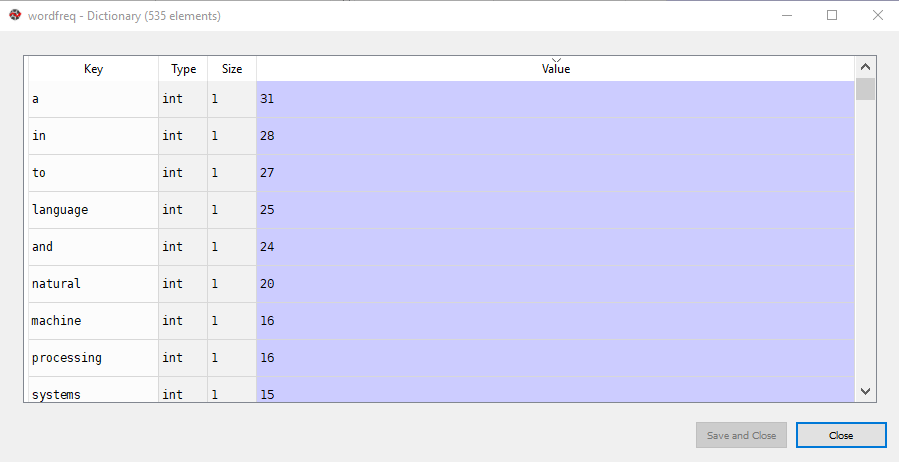You can see words in the “Key” column and their frequency of occurrences in the “Value” column.

As I said in the theory section, depending upon the task at hand, not all of the words are useful. In huge corpora, you can have millions of words. We can filter the most frequently occurring words. Our corpus has 535 words in total. Let us filter down to the 200 most frequently occurring words. To do so, we can make use of Python’s `heap` library.

Look at the following script:

``import heapq   most_freq = heapq.nlargest(200, wordfreq, key=wordfreq.get)   ``

Now our `most_freq` list contains 200 most frequently occurring words along with their frequency of occurrence.

The final step is to convert the sentences in our corpus into their corresponding vector representation. The idea is straightforward, for each word in the `most_freq` dictionary if the word exists in the sentence, a 1 will be added for the word, else 0 will be added.

``sentence_vectors = []   for sentence in corpus:       sentence_tokens = nltk.word_tokenize(sentence)     sent_vec = []     for token in most_freq:         if token in sentence_tokens:             sent_vec.append(1)         else:             sent_vec.append(0)     sentence_vectors.append(sent_vec) ``

In the script above we create an empty list `sentence_vectors` which will store vectors for all the sentences in the corpus. Next, we iterate through each sentence in the corpus and create an empty list `sent_vec` for the individual sentences. Similarly, we also tokenize the sentence. Next, we iterate through each word in the `most_freq` list and check if the word exists in the tokens for the sentence. If the word is a part of the sentence, 1 is appended to the individual sentence vector `sent_vec`, else 0 is appended. Finally, the sentence vector is added to the list `sentence_vectors` which contains vectors for all the sentences. Basically, this `sentence_vectors` is our bag of words model.

However, the bag of words model that we saw in the theory section was in the form of a matrix. Our model is in the form of a list of lists. We can convert our model into matrix form using this script:

``sentence_vectors = np.asarray(sentence_vectors)   ``

Basically, in the following script, we converted our list into a two-dimensional numpy array using `asarray` function. Now if you open the `sentence_vectors` variable in the variable explorer of the Spyder editor, you should see the following matrix: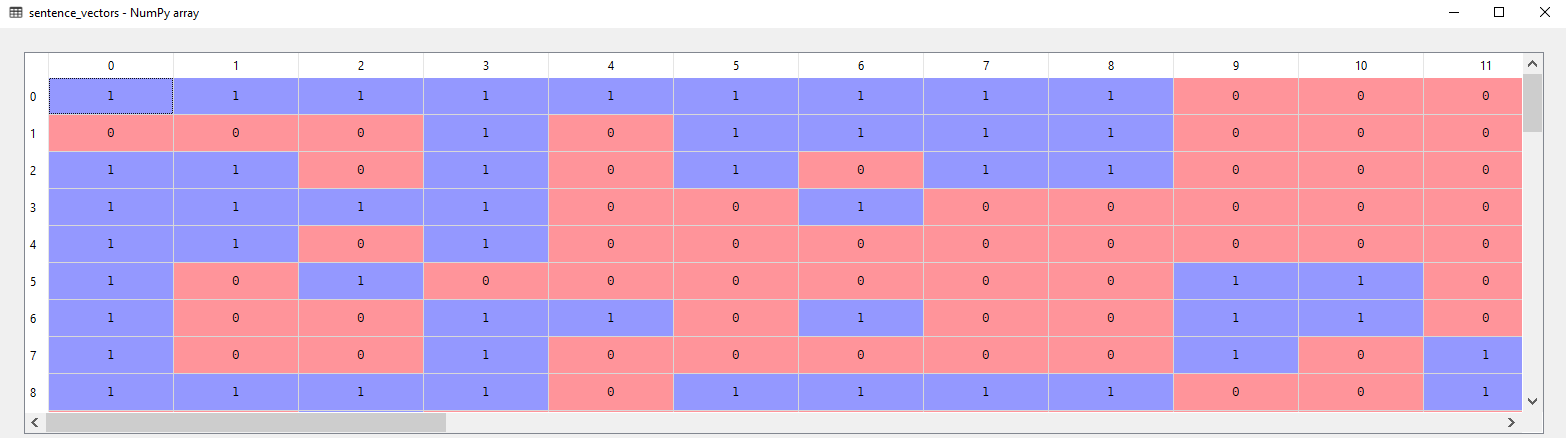You can see the Bag of Words model containing 0 and 1.

### Conclusion

Bag of Words model is one of the three most commonly used word embedding approaches with TF-IDF and Word2Vec being the other two.

In this article, we saw how to implement the Bag of Words approach from scratch in Python. The theory of the approach has been explained along with the hands-on code to implement the approach. In the next article, we will see how to implement the TF-IDF approach from scratch in Python.

Planet Python

## Stack Abuse: Text Generation with Python and TensorFlow/Keras

### Introduction

﻿Are you interested in using a neural network to generate text? TensorFlow and Keras can be used for some amazing applications of natural language processing techniques, including the generation of text.

In this tutorial, we’ll cover the theory behind text generation using a Recurrent Neural Networks, specifically a Long Short-Term Memory Network, implement this network in Python, and use it to generate some text.

### Defining Terms

To begin with, let’s start by defining our terms. It may prove difficult to understand why certain lines of code are being executed unless you have a decent understanding of the concepts that are being brought together.

#### TensorFlow

TensorFlow is one of the most commonly used machine learning libraries in Python, specializing in the creation of deep neural networks. Deep neural networks excel at tasks like image recognition and recognizing patterns in speech. TensorFlow was designed by Google Brain, and its power lies in its ability to join together many different processing nodes.

#### Keras

Meanwhile, Keras is an application programming interface or API. Keras makes use of TensorFlow’s functions and abilities, but it streamlines the implementation of TensorFlow functions, making building a neural network much simpler and easier. Keras’ foundational principles are modularity and user-friendliness, meaning that while Keras is quite powerful, it is easy to use and scale.

#### Natural Language Processing

Natural Language Processing (NLP) is exactly what it sounds like, the techniques used to enable computers to understand natural human language, rather than having to interface with people through programming languages. Natural language processing is necessary for tasks like the classification of word documents or the creation of a chatbot.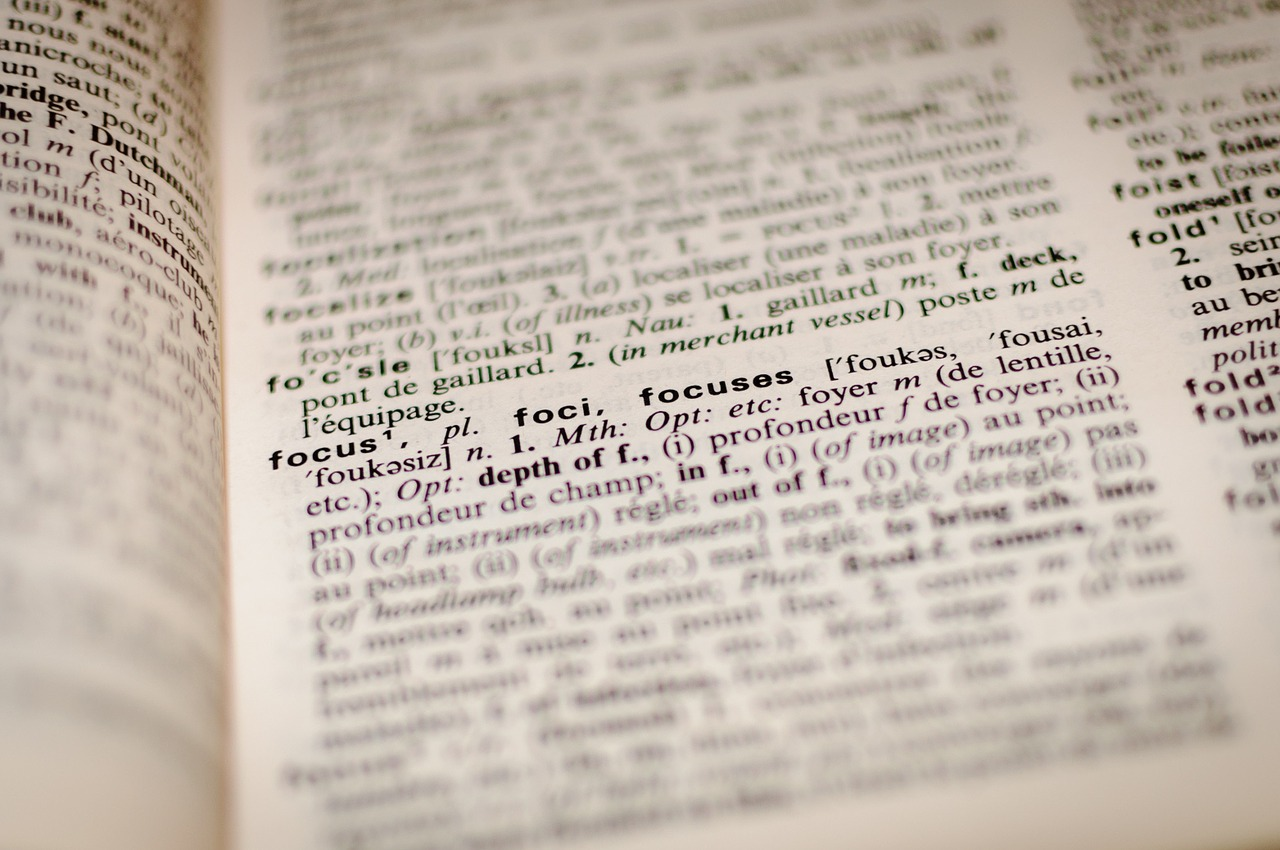#### Corpus

A corpus is a large collection of text, and in the machine learning sense a corpus can be thought of as your model’s input data. The corpus contains the text you want the model to learn about.

It is common to divide a large corpus into training and testing sets, using most of the corpus to train the model on and some unseen part of the corpus to test the model on, although the testing set can be an entirely different set of data. The corpus typically requires preprocessing to become fit for usage in a machine learning system.

#### Encoding

Encoding is sometimes referred to as word representation and it refers to the process of converting text data into a form that a machine learning model can understand. Neural networks cannot work with raw text data, the characters/words must be transformed into a series of numbers the network can interpret.

The actual process of converting words into number vectors is referred to as “tokenization”, because you obtain tokens that represent the actual words. There are multiple ways to encode words as number values. The primary methods of encoding are one-hot encoding and creating densely embedded vectors.

We’ll go into the difference between these methods in the theory section below.

#### Recurrent Neural Network

A basic neural network links together a series of neurons or nodes, each of which take some input data and transform that data with some chosen mathematical function. In a basic neural network, the data has to be a fixed size, and at any given layer in the neural network the data being passed in is simply the outputs of the previous layer in the network, which are then transformed by the weights for that layer.

In contrast, a Recurrent Neural Network differs from a “vanilla” neural network thanks to its ability to remember prior inputs from previous layers in the neural network.

To put that another way, the outputs of layers in a Recurrent Neural Network aren’t influenced only by the weights and the output of the previous layer like in a regular neural network, but they are also influenced by the “context” so far, which is derived from prior inputs and outputs.

Recurrent Neural Networks are useful for text processing because of their ability to remember the different parts of a series of inputs, which means that they can take the previous parts of a sentence into account to interpret context.

#### Long Short-Term Memory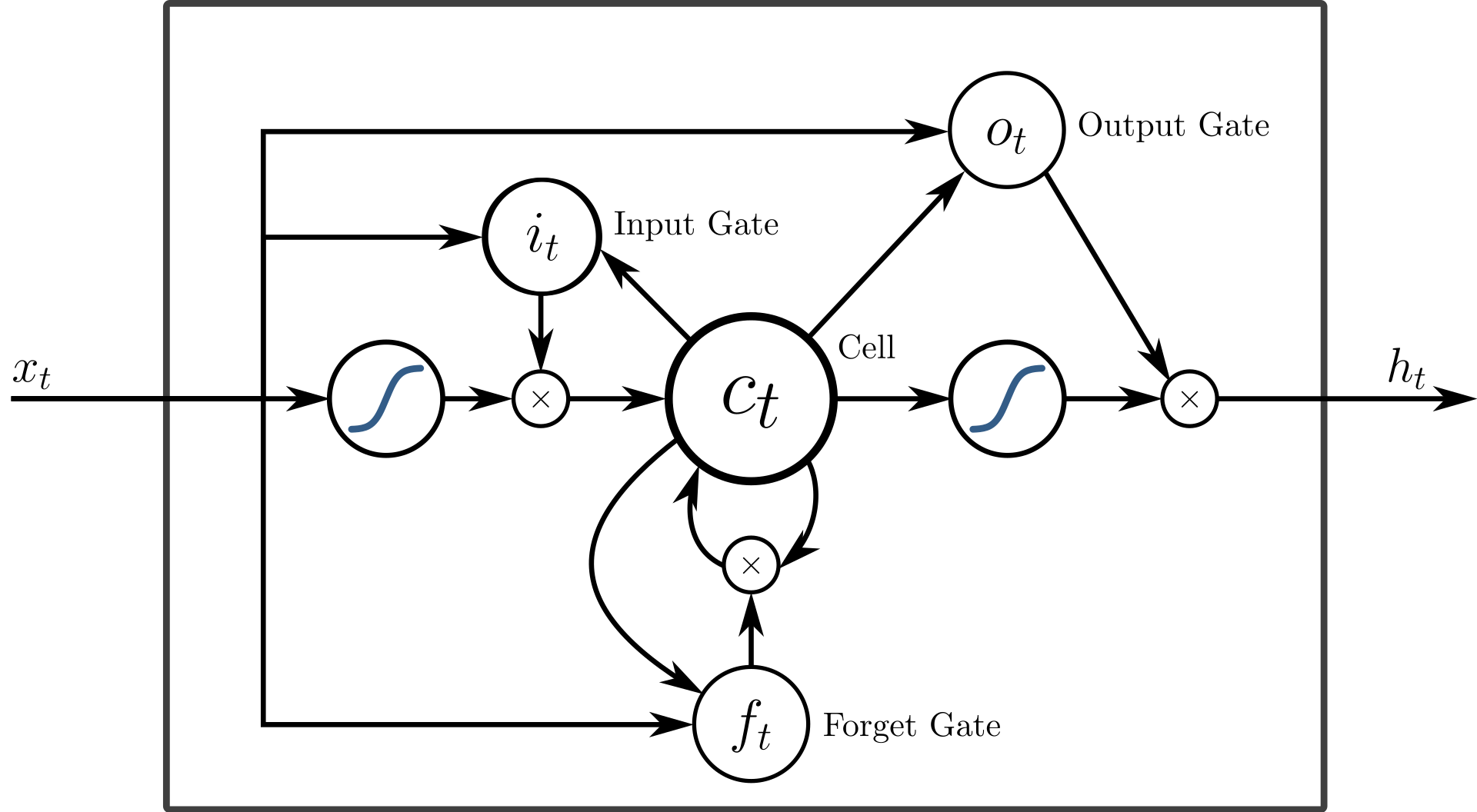Long Short-Term Memory (LSTMs) networks are a specific type of Recurrent Neural Networks. LSTMs have advantages over other recurrent neural networks. While recurrent neural networks can usually remember previous words in a sentence, their ability to preserve the context of earlier inputs degrades over time.

The longer the input series is, the more the network “forgets”. Irrelevant data is accumulated over time and it blocks out the relevant data needed for the network to make accurate predictions about the pattern of the text. This is referred to as the vanishing gradient problem.

You don’t need to understand the algorithms that deal with the vanishing gradient problem (although you can read more about it here), but know that an LSTM can deal with this problem by selectively “forgetting” information deemed nonessential to the task at hand. By suppressing nonessential information, the LSTM is able to focus on only the information that genuinely matters, taking care of the vanishing gradient problem. This makes LSTMs more robust when handling long strings of text.

### Text Generation Theory/Approach

#### Encoding Revisited

##### One-Hot Encoding

As previously mentioned, there are two main ways of encoding text data. One method is referred to as one-hot encoding, while the other method is called word embedding.

The process of one-hot encoding refers to a method of representing text as a series of ones and zeroes. A vector containing all possible words you are interested in, often all the words in the corpus, is created and a single word is represented by a “one” value in its respective position. Meanwhile all other positions (all the other possible words) are given a zero value. A vector like this is created for every word in the feature set, and when the vectors are joined together the result is a matrix containing binary representations of all the feature words.

Here’s another way to think about this: any given word is represented by a vector of ones and zeroes, with a one value at a unique position. The vector is essentially concerned with answering the question: “Is this the target word?” If the word in the list of feature words is the target a positive value (one) is entered there, and in all other cases the word isn’t the target, so a zero is entered. Therefore, you have a vector that represents just the target word. This is done for every word in the list of features.

One-hot encodings are useful when you need to need to create a bag of words, or a representation of words that takes their frequency of occurrence into account. Bag of words models are useful because although they are simple models, they still maintain a lot of important information and are versatile enough to be used for many different NLP related tasks.

One drawback to using one-hot encodings is that they cannot represent the meaning of a word, nor can they easily detect similarities between words. If meaning and similarity are concerns, word embeddings are often used instead.

##### Word Embeddings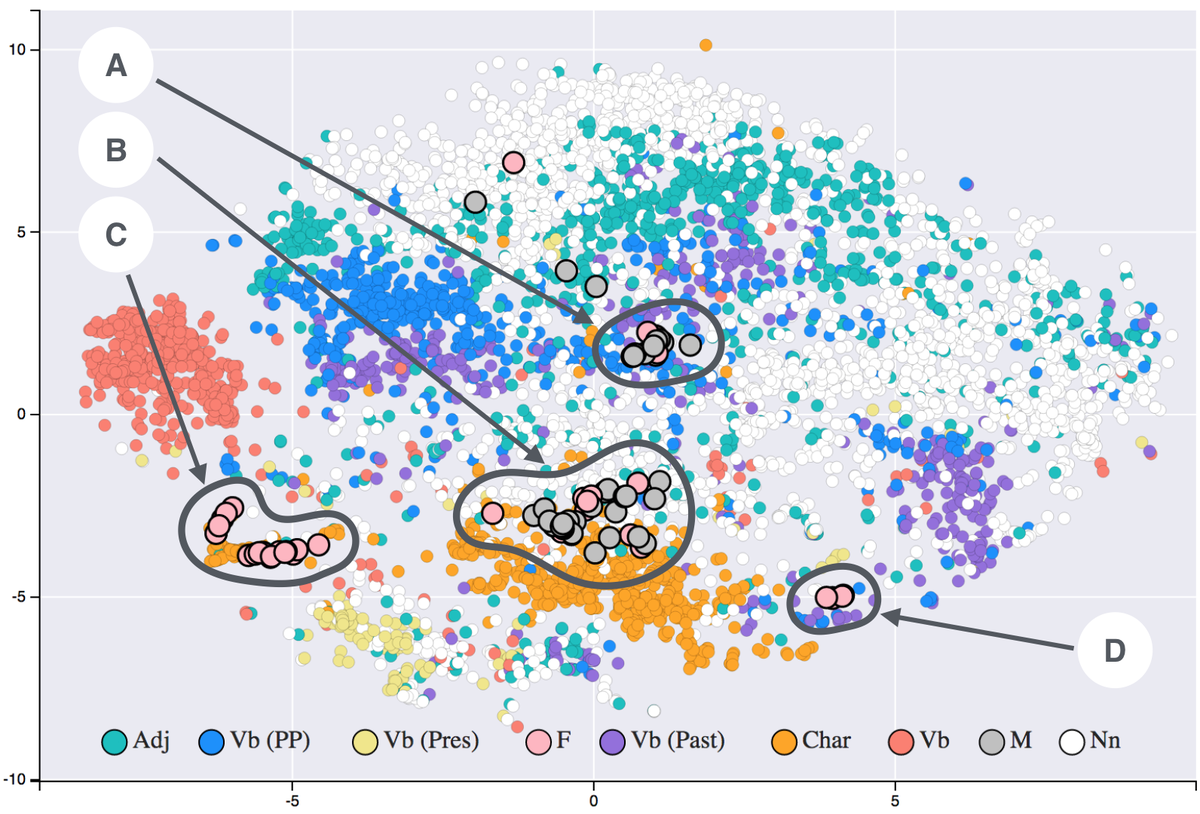Word embedding refers to representing words or phrases as a vector of real numbers, much like one-hot encoding does. However, a word embedding can use more numbers than simply ones and zeros, and therefore it can form more complex representations. For instance, the vector that represents a word can now be comprised of decimal values like 0.5. These representations can store important information about words, like relationship to other words, their morphology, their context, etc.

Word embeddings have fewer dimensions than one-hot encoded vectors do, which forces the model to represent similar words with similar vectors. Each word vector in a word embedding is a representation in a different dimension of the matrix, and the distance between the vectors can be used to represent their relationship. Word embeddings can generalize because semantically similar words have similar vectors. The word vectors occupy a similar region of the matrix, which helps capture context and semantics.

In general, one-hot vectors are high-dimensional but sparse and simple, while word embeddings are low dimensional but dense and complex.

#### Word-Level Generation vs Character-Level Generation

There are two ways to tackle a natural language processing task like text generation. You can analyze the data and make predictions about it at the level of the words in the corpus or at the level of the individual characters. Both character-level generation and word-level generation have their advantages and disadvantages.

In general, word-level language models tend to display higher accuracy than character-level language models. This is because they can form shorter representations of sentences and preserve the context between words easier than character-level language models. However, large corpuses are needed to sufficiently train word-level language models, and one-hot encoding isn’t very feasible for word level models.

In contrast, character-level language models are often quicker to train, requiring less memory and having faster inference than word-based models. This is because the “vocabulary” (the number of training features) for the model is likely to be much smaller overall, limited to some hundreds of characters rather than hundreds of thousands of words.

Character-based models also perform well when translating words between languages because they capture the characters which make up words, rather than trying to capture the semantic qualities of words. We’ll be using a character-level model here, in part because of its simplicity and fast inference.

#### Using an RNN/LSTM

When it comes to implementing an LSTM in Keras, the process is similar to implementing other neural networks created with the sequential model. You start by declaring the type of model structure you are going to use, and then add layers to the model one at a time. LSTM layers are readily accessible to us in Keras, we just have to import the layers and then add them with `model.add`.

In between the primary layers of the LSTM, we will use layers of dropout, which helps prevent the issue of overfitting. Finally, the last layer in the network will be a densely connected layer that will use a sigmoid activation function and output probabilities.

#### Sequences and Features

It is important to understand how we will be handling our input data for our model. We will be dividing the input words into chunks and sending these chunks through the model one at a time.

The features for our model are simply the words we are interested in analyzing, as represented with the bag of words. The chunks that we divide the corpus into are going to be sequences of words, and you can think of every sequence as an individual training instance/example in a traditional machine learning task.

### Implementing an LSTM for Text Generation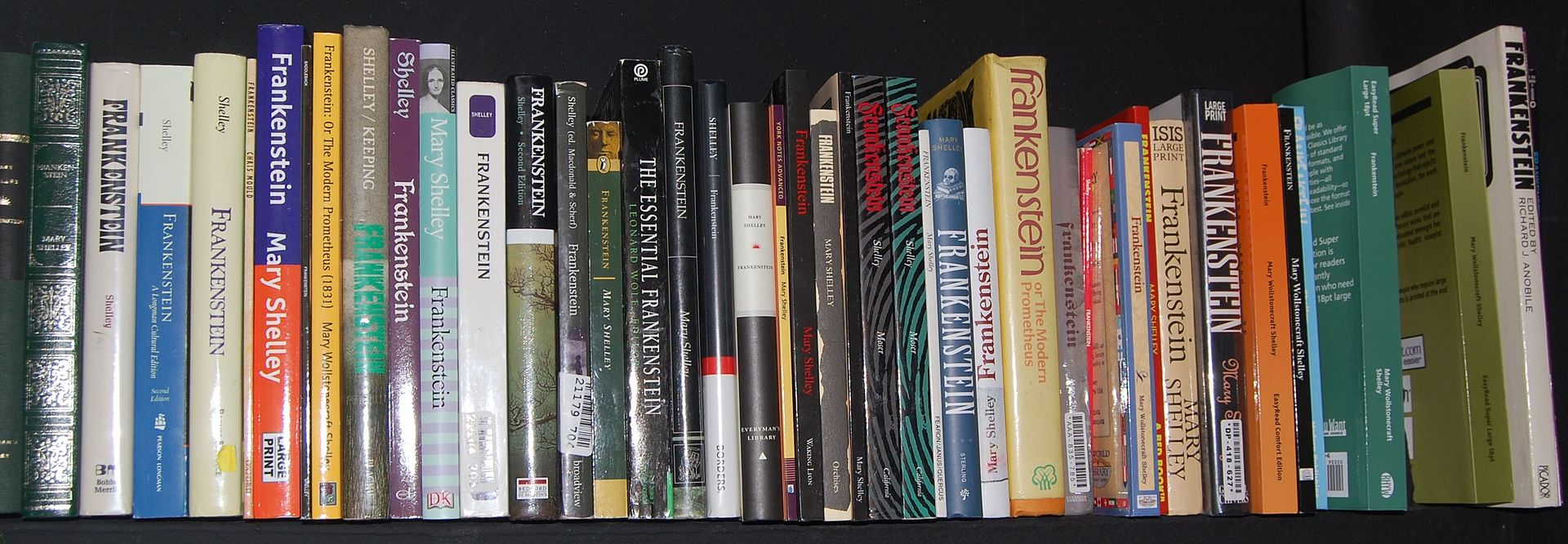Now we’ll be implementing a LSTM and doing text generation with it. First, we’ll need to get some text data and preprocess the data. After that, we’ll create the LSTM model and train it on the data. Finally, we’ll evaluate the network.

For the text generation, we want our model to learn probabilities about what character will come next, when given a starting (random) character. We will then chain these probabilities together to create an output of many characters. We first need to convert our input text to numbers and then train the model on sequences of these numbers.

Let’s start out by importing all the libraries we’re going to use. We need `numpy` to transform our input data into arrays our network can use, and we’ll obviously be using several functions from Keras.

We’ll also need to use some functions from the Natural Language Toolkit (NLTK) to preprocess our text and get it ready to train on. Finally, we’ll need the `sys` library to handle the printing of our text:

``import numpy   import sys   from nltk.tokenize import RegexpTokenizer   from nltk.corpus import stopwords   from keras.models import Sequential   from keras.layers import Dense, Dropout, LSTM   from keras.utils import np_utils   from keras.callbacks import ModelCheckpoint   ``

To start off with, we need to have data to train our model on. You can use any text file you’d like for this, but we’ll be using part of Mary Shelley’s Frankenstein, which is available for download at Project Gutenburg, which hosts public domain texts.

We’ll be training the network on the text from the first 9 chapters:

``file = open("frankenstein-2.txt").read()   ``

Let’s start by loading in our text data and doing some preprocessing of the data. We’re going to need to apply some transformations to the text so everything is standardized and our model can work with it.

We’re going to lowercase everything so and not worry about capitalization in this example. We’re also going to use NLTK to make tokens out of the words in the input file. Let’s create an instance of the tokenizer and use it on our input file.

Finally, we’re going to filter our list of tokens and only keep the tokens that aren’t in a list of Stop Words, or common words that provide little information about the sentence in question. We’ll do this by using `lambda` to make a quick throwaway function and only assign the words to our variable if they aren’t in a list of Stop Words provided by NLTK.

Let’s create a function to handle all that:

``def tokenize_words(input):       # lowercase everything to standardize it     input = input.lower()      # instantiate the tokenizer     tokenizer = RegexpTokenizer(r'\w+')     tokens = tokenizer.tokenize(input)      # if the created token isn't in the stop words, make it part of "filtered"     filtered = filter(lambda token: token not in stopwords.words('english'), tokens)     return " ".join(filtered) ``

Now we call the function on our file:

``# preprocess the input data, make tokens processed_inputs = tokenize_words(file)   ``

A neural network works with numbers, not text characters. So well need to convert the characters in our input to numbers. We’ll sort the list of the set of all characters that appear in our input text, then use the `enumerate` function to get numbers which represent the characters. We then create a dictionary that stores the keys and values, or the characters and the numbers that represent them:

``chars = sorted(list(set(processed_inputs)))   char_to_num = dict((c, i) for i, c in enumerate(chars))   ``

We need the total length of our inputs and total length of our set of characters for later data prep, so we’ll store these in a variable. Just so we get an idea of if our process of converting words to characters has worked thus far, let’s print the length of our variables:

``input_len = len(processed_inputs)   vocab_len = len(chars)   print ("Total number of characters:", input_len)   print ("Total vocab:", vocab_len)   ``

Here’s the output:

``Total number of characters: 100581   Total vocab: 42   ``

Now that we’ve transformed the data into the form it needs to be in, we can begin making a dataset out of it, which we’ll feed into our network. We need to define how long we want an individual sequence (one complete mapping of inputs characters as integers) to be. We’ll set a length of 100 for now, and declare empty lists to store our input and output data:

``seq_length = 100   x_data = []   y_data = []   ``

Now we need to go through the entire list of inputs and convert the characters to numbers. We’ll do this with a `for` loop. This will create a bunch of sequences where each sequence starts with the next character in the input data, beginning with the first character:

``# loop through inputs, start at the beginning and go until we hit # the final character we can create a sequence out of for i in range(0, input_len - seq_length, 1):       # Define input and output sequences     # Input is the current character plus desired sequence length     in_seq = processed_inputs[i:i + seq_length]      # Out sequence is the initial character plus total sequence length     out_seq = processed_inputs[i + seq_length]      # We now convert list of characters to integers based on     # previously and add the values to our lists     x_data.append([char_to_num[char] for char in in_seq])     y_data.append(char_to_num[out_seq]) ``

Now we have our input sequences of characters and our output, which is the character that should come after the sequence ends. We now have our training data features and labels, stored as `x_data` and `y_data.` Let’s save our total number of sequences and check to see how many total input sequences we have:

``n_patterns = len(x_data)   print ("Total Patterns:", n_patterns)   ``

Here’s the output:

``Total Patterns: 100481   ``

Now we’ll go ahead and convert our input sequences into a processed numpy array that our network can use. We’ll also need to convert the numpy array values into floats so that the sigmoid activation function our network uses can interpret them and output probabilities from 0 to 1:

``X = numpy.reshape(x_data, (n_patterns, seq_length, 1))   X = X/float(vocab_len)   ``

We’ll now one-hot encode our label data:

``y = np_utils.to_categorical(y_data)   ``

Since our features and labels are now ready for the network to use, let’s go ahead and create our LSTM model. We specify the kind of model we want to make (a `sequential` one), and then add our first layer.

We’ll do dropout to prevent overfitting, followed by another layer or two. Then we’ll add the final layer, a densely connected layer that will output a probability about what the next character in the sequence will be:

``model = Sequential()   model.add(LSTM(256, input_shape=(X.shape, X.shape), return_sequences=True))   model.add(Dropout(0.2))   model.add(LSTM(256, return_sequences=True))   model.add(Dropout(0.2))   model.add(LSTM(128))   model.add(Dropout(0.2))   model.add(Dense(y.shape, activation='softmax'))   ``

We compile the model now, and it is ready for training:

``model.compile(loss='categorical_crossentropy', optimizer='adam')   ``

It takes the model quite a while to train, and for this reason we’ll save the weights and reload them when the training is finished. We’ll set a `checkpoint` to save the weights to, and then make them the callbacks for our future model.

``filepath = "model_weights_saved.hdf5"   checkpoint = ModelCheckpoint(filepath, monitor='loss', verbose=1, save_best_only=True, mode='min')   desired_callbacks = [checkpoint]   ``

Now we’ll fit the model and let it train.

``model.fit(X, y, epochs=4, batch_size=256, callbacks=desired_callbacks)   ``

After it has finished training, we’ll specify the file name and load in the weights. Then recompile our model with the saved weights:

``filename = "model_weights_saved.hdf5"   model.load_weights(filename)   model.compile(loss='categorical_crossentropy', optimizer='adam')   ``

Since we converted the characters to numbers earlier, we need to define a dictionary variable that will convert the output of the model back into numbers:

``num_to_char = dict((i, c) for i, c in enumerate(chars))   ``

To generate characters, we need to provide our trained model with a random seed character that it can generate a sequence of characters from:

``start = numpy.random.randint(0, len(x_data) - 1)   pattern = x_data[start]   print("Random Seed:")   print("\"", ''.join([num_to_char[value] for value in pattern]), "\"")   ``

Here’s an example of a random seed:

``" ed destruction pause peace grave succeeded sad torments thus spoke prophetic soul torn remorse horro " ``

Now to finally generate text, we’re going to iterate through our chosen number of characters and convert our input (the random seed) into `float` values.

We’ll ask the model to predict what comes next based off of the random seed, convert the output numbers to characters and then append it to the pattern, which is our list of generated characters plus the initial seed:

``for i in range(1000):       x = numpy.reshape(pattern, (1, len(pattern), 1))     x = x / float(vocab_len)     prediction = model.predict(x, verbose=0)     index = numpy.argmax(prediction)     result = num_to_char[index]     seq_in = [num_to_char[value] for value in pattern]      sys.stdout.write(result)      pattern.append(index)     pattern = pattern[1:len(pattern)] ``

Let’s see what it generated.

``"er ed thu so sa fare ver ser ser er serer serer serer serer serer serer serer serer serer serer serer serer serer serer serer serer serer serer...." ``

Does this seem somewhat disappointing? Yes, the text that was generated doesn’t make any sense, and it seems to start simply repeating patterns after a little bit. However, the longer you train the network the better the text that is generated will be.

For instance, when the number of training epochs was increased to 20, the output looked more like this:

``"ligther my paling the same been the this manner to the forter the shempented and the had an ardand the verasion the the dears conterration of the astore" ``

The model is now generating actual words, even if most of it still doesn’t make sense. Still, for only around 100 lines of code, it isn’t bad.

Now you can play around with the model yourself and try adjusting the parameters to get better results.

### Conclusion

You’ll want to increase the number of training epochs to improve the network’s performance. However, you may also want to use either a deeper neural network (add more layers to the network) or a wider network (increase the number of neurons/memory units) in the layers.

You could also try adjusting the batch size, one hot-encoding the inputs, padding the input sequences, or combining any number of these ideas.

If you want to learn more about how LSTMs work, you can read up on the subject here. Learning how the parameters of the model influence the model’s performance will help you choose which parameters or hyperparameters to adjust. You may also want to read up on text processing techniques and tools like those provided by NLTK.

If you’d like to read more about Natural Language Processing in Python, we’ve got a 12-part series that goes in-depth: Python for NLP.

You can also look at other implementations of LSTM text generation for ideas, such as Andrej Karpathy’s blog post, which is one of the most famous uses of an LSTM to generate text.

Planet Python